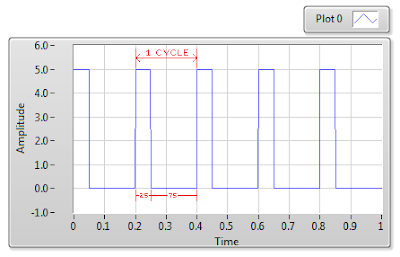Pulse Width Modulation is one of the important functions of timer. PWM is a technique to control analogue circuits with digital output from microcontroller.

As you all know that an Analogue signal is the one whose value varies continuously with time whereas digital signals can only be either high or low. PWM is used for generating an analogue signal using a digital source.

PWM consist of two main components:-
1.) Duty cycle
2.) Frequency

Duty cycle describes the amount of time, the signal is in HIGH state as a percentage of total time, it takes to complete one cycle. Frequency describes how fast the PWM completes a cycle and therefore how fast it switches between HIGH and LOW.

By cycling a digital signal ON and OFF at a fast enough rate and at a certain duty cycle, the output will appear like a constant voltage analogue signal. For eg: To create a 2V signal from a digital source which is either HIGH(5V) or low (0). We can use PWM with duty cycle of 40% here. This 40% duty cycle would yield an average voltage of 5x0.4=2V.DUTY CYCLE OF 25%DUTY CYCLE OF 75%

I am going to use PWM to vary the brightness of a LED by using an input button. SO on each press of the button, the duty cycle will vary from 0 to 100% in 10 steps.  After 100% , it will go back to 0.
The following is my setup of STM32CubeMx:

First we need to select PWM in the timer section. Also see the PIN which is connected to respective channel of the PWM. PC13 is being used as an input button with internal PULL-UP.

In the CONFIGURATION tab, select timer and make sure your setup is as above. 84-1 will divide the clock frequency by 84, making it 1 MHz. 100-1 further divides the clock. It is the value for ARR( Auto reload Register), The counter counts upto this value and than reset back to 0. So making it 100 is more preferred as it can be counted as %. For eg if the pulse is set for 30 and ARR is 100, it indicates that the duty cycle is 30%.

The clock division is (84MHz/84)/100) giving us 10KHz frequency.
Also 100-1 and 84-1 are such that  HAL adds 1 to these values so we have to write 1 less than the value we want.
This is all regarding the CubeMx setup. Now generate the project and open the IDE.

### Some Insight into the Code:-

``````if (!(HAL_GPIO_ReadPin (GPIOC, GPIO_PIN_13)))   // if the user button is pressed
{
while (!(HAL_GPIO_ReadPin (GPIOC, GPIO_PIN_13)));  // wait for the button to be released
htim3.Instance->CCR1 = increment;   // increase the pulse width in the steps of 10
increment+=10;  // increment in 10 steps or by 10% for the duty cycle if (increment>100)
increment =0;   // if increment is > 100 than reset its value to 0
}
``````

CHECK OUT THE VIDEO BELOW

PWM (Pulse Width Mod) in STM32Reviewed by arun singh on January 14, 2018 Rating: 5